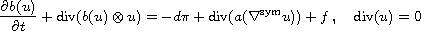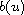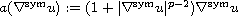Variational and Topological Methods: Theory, Applications, Numerical Simulations, and Open Problems.
Electron. J. Diff. Eqns., Conference 21 (2014), pp. 223-234.

### Extinction of weak solutions of doubly nonlinear Navier-Stokes equations Jochen Merker

Abstract:
In this article we discus the doubly nonlinear incompressible Navier-Stokes equationsare discussed, where u models the velocity vector field of a homogeneous incompressible non-Newtonian fluid whose momentumdepends nonlinearly on u. Particularly, under certain regularity assumptions it is shown that u becomes extinct in finite time for sufficiently small initial values u(0), ifandwithI.

Published February 10, 2014.
Math Subject Classifications: 58F15, 58F17, 53C35.
Key Words: Navier-Stokes equations; doubly nonlinear evolution equations; extinction.

Show me the PDF(287 K), TEX and other files for this article.Jochen Merker Universitäat Rostock - Institut für Mathematik Ulmenstr. 69 (Haus 3) 18057 Rostock, Germany email: jochen.merker@uni-rostock.de